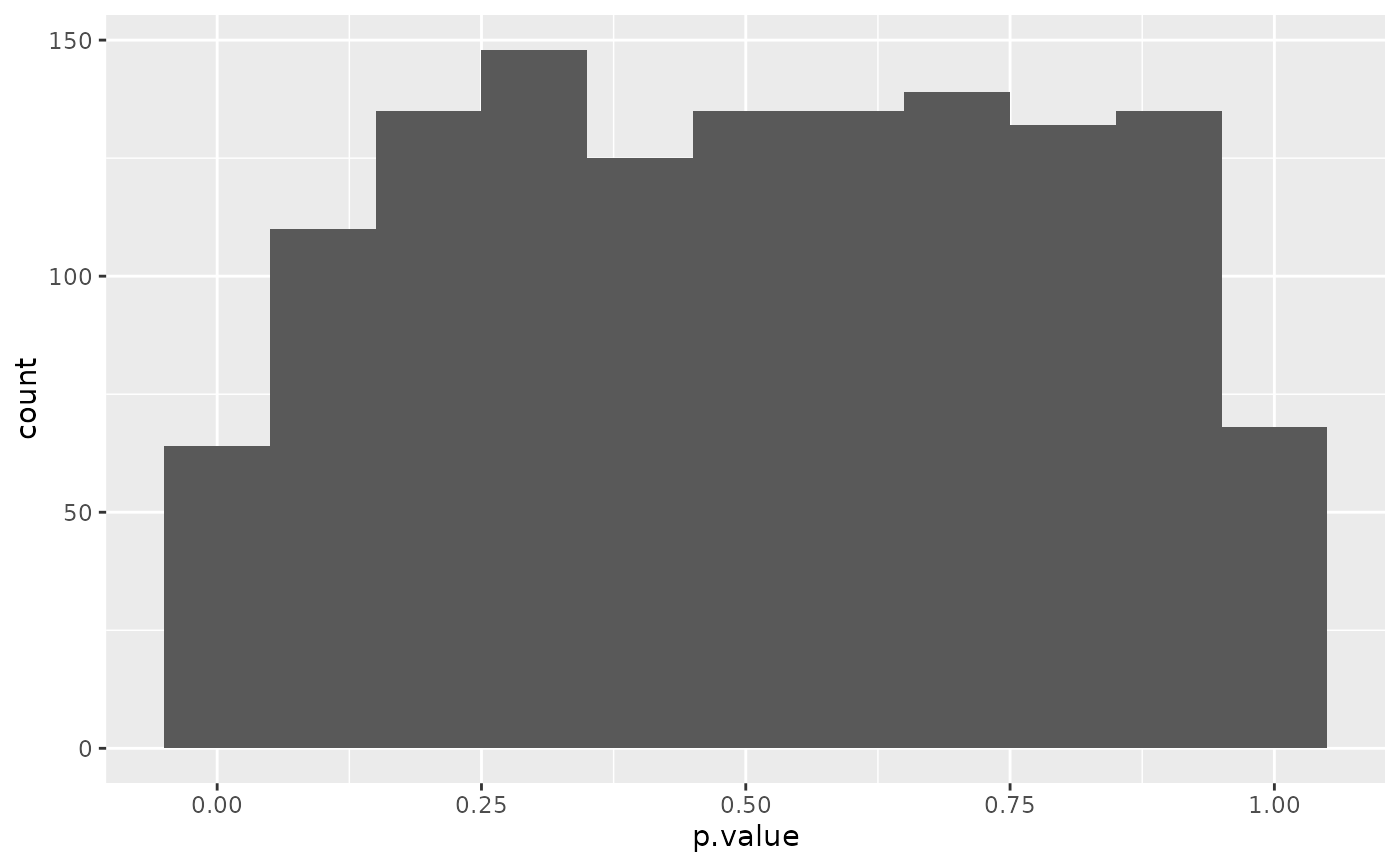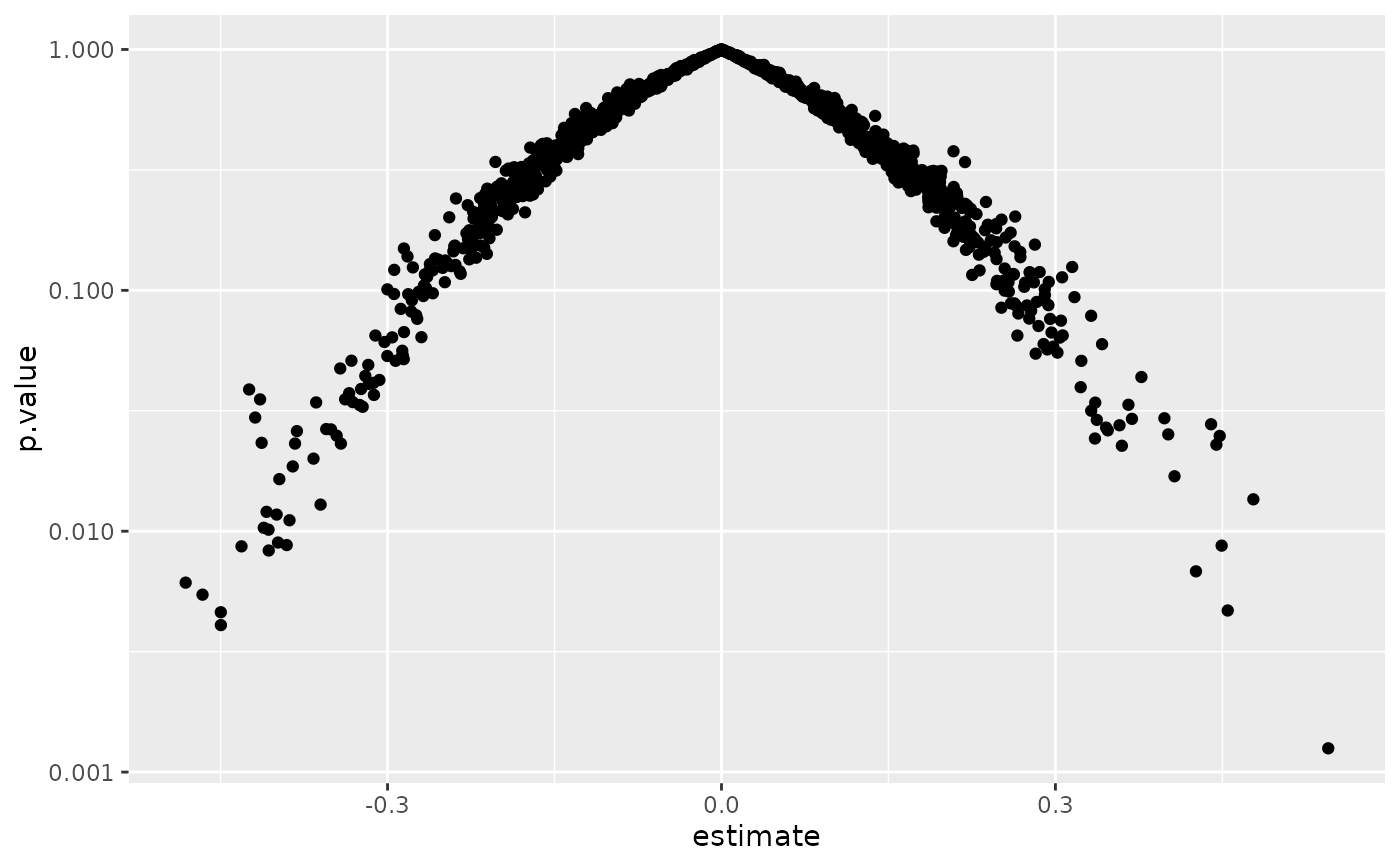Tidy summarizes information about the components of a model. A model component might be a single term in a regression, a single hypothesis, a cluster, or a class. Exactly what tidy considers to be a model component varies across models but is usually self-evident. If a model has several distinct types of components, you will need to specify which components to return.

## Usage

# S3 method for rcorr
tidy(x, diagonal = FALSE, ...)

## Arguments

x

An rcorr object returned from Hmisc::rcorr().

diagonal

Logical indicating whether or not to include diagonal elements of the correlation matrix, or the correlation of a column with itself. For the elements, estimate is always 1 and p.value is always NA. Defaults to FALSE.

...

Additional arguments. Not used. Needed to match generic signature only. Cautionary note: Misspelled arguments will be absorbed in ..., where they will be ignored. If the misspelled argument has a default value, the default value will be used. For example, if you pass conf.lvel = 0.9, all computation will proceed using conf.level = 0.95. Two exceptions here are:

• tidy() methods will warn when supplied an exponentiate argument if it will be ignored.

• augment() methods will warn when supplied a newdata argument if it will be ignored.

## Details

Suppose the original data has columns A and B. In the correlation matrix from rcorr there may be entries for both the cor(A, B) and cor(B, A). Only one of these pairs will ever be present in the tidy output.

tidy(), Hmisc::rcorr()

## Value

A tibble::tibble() with columns:

column1

Name or index of the first column being described.

column2

Name or index of the second column being described.

estimate

The estimated value of the regression term.

p.value

The two-sided p-value associated with the observed statistic.

n

Number of observations used to compute the correlation

## Examples


# load libraries for models and data
library(Hmisc)
#>
#> Attaching package: ‘Hmisc’
#> The following object is masked from ‘package:psych’:
#>
#>     describe
#> The following object is masked from ‘package:network’:
#>
#>     is.discrete
#> The following object is masked from ‘package:survey’:
#>
#>     deff
#> The following object is masked from ‘package:quantreg’:
#>
#>     latex
#> The following objects are masked from ‘package:dplyr’:
#>
#>     src, summarize
#> The following objects are masked from ‘package:base’:
#>
#>     format.pval, units

mat <- replicate(52, rnorm(100))

mat[sample(length(mat), 2000)] <- NA

# also, column names
colnames(mat) <- c(LETTERS, letters)

# fit model
rc <- rcorr(mat)

# summarize model fit with tidiers  + visualization
td <- tidy(rc)
td
#> # A tibble: 1,326 × 5
#>    column1 column2 estimate     n p.value
#>    <chr>   <chr>      <dbl> <int>   <dbl>
#>  1 B       A        -0.0806    41 0.616
#>  2 C       A        -0.194     38 0.242
#>  3 C       B         0.0811    37 0.633
#>  4 D       A        -0.451     37 0.00505
#>  5 D       B        -0.258     35 0.134
#>  6 D       C        -0.183     35 0.292
#>  7 E       A        -0.0593    42 0.709
#>  8 E       B         0.0208    45 0.892
#>  9 E       C        -0.228     44 0.136
#> 10 E       D        -0.0134    34 0.940
#> # ℹ 1,316 more rows

library(ggplot2)
ggplot(td, aes(p.value)) +
geom_histogram(binwidth = .1)ggplot(td, aes(estimate, p.value)) +
geom_point() +
scale_y_log10()